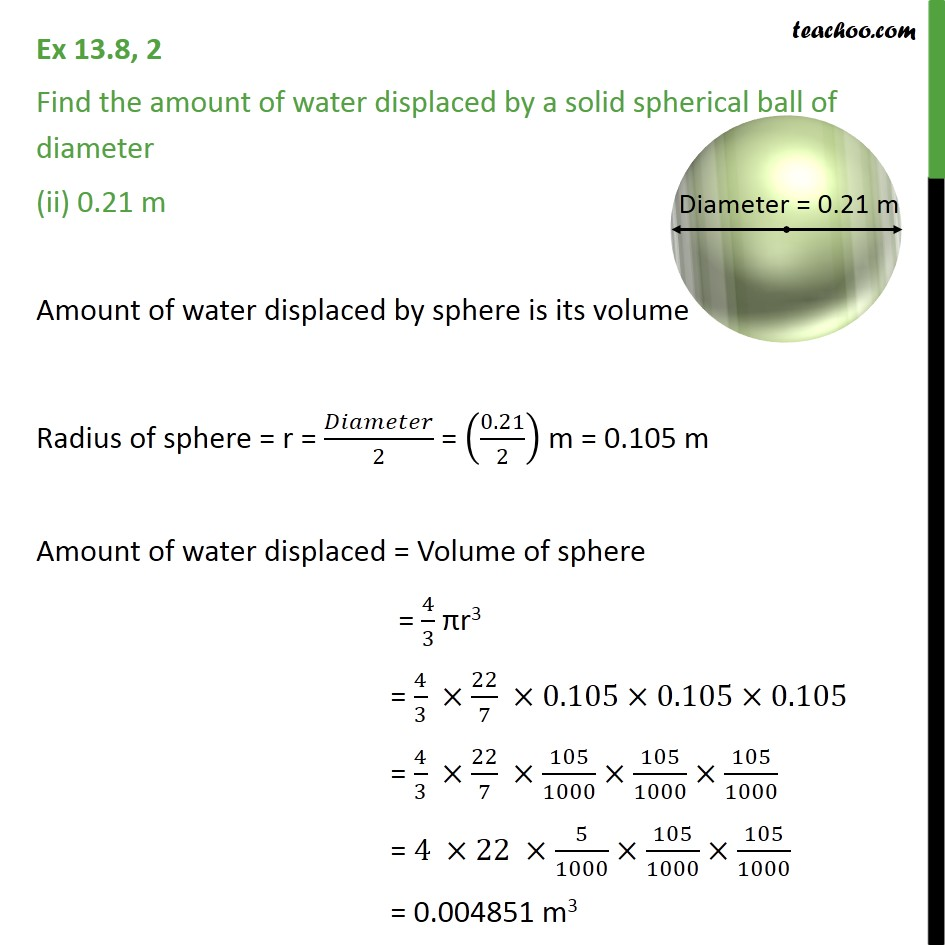Ex 11.4

Chapter 11 Class 9 Surface Areas and Volumes
Serial order wiseLearn in your speed, with individual attention - Teachoo Maths 1-on-1 Class

### Transcript

Ex 11.4, 2 Find the amount of water displaced by a solid spherical ball of diameter (ii) 0.21 m Amount of water displaced by sphere is its volume Radius of sphere = r = /2 = (0.21/2) m = 0.105 m Amount of water displaced = Volume of sphere = 4/3 r3 = 4/3 22/7 0.105 0.105 0.105 = 4/3 22/7 105/1000 105/1000 105/1000 = 4 22 5/1000 105/1000 105/1000 = 0.004851 m3